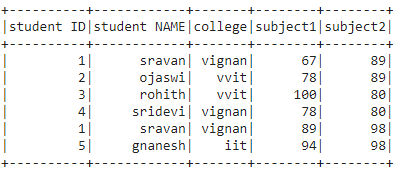Related Articles

# Convert PySpark dataframe to list of tuples

• Last Updated : 18 Jul, 2021

In this article, we are going to convert the Pyspark dataframe into a list of tuples.

The rows in the dataframe are stored in the list separated by a comma operator. So we are going to create a dataframe by using a nested list

Creating Dataframe for demonstration:

## Python3

 `# importing module``import` `pyspark`` ` `# importing sparksession from pyspark.sql module``from` `pyspark.sql ``import` `SparkSession`` ` `# creating sparksession and giving an app name``spark ``=` `SparkSession.builder.appName(``'sparkdf'``).getOrCreate()`` ` `# list  of students  data``data ``=` `[[``"1"``, ``"sravan"``, ``"vignan"``, ``67``, ``89``],``        ``[``"2"``, ``"ojaswi"``, ``"vvit"``, ``78``, ``89``],``        ``[``"3"``, ``"rohith"``, ``"vvit"``, ``100``, ``80``],``        ``[``"4"``, ``"sridevi"``, ``"vignan"``, ``78``, ``80``],``        ``[``"1"``, ``"sravan"``, ``"vignan"``, ``89``, ``98``],``        ``[``"5"``, ``"gnanesh"``, ``"iit"``, ``94``, ``98``]]`` ` `# specify column names``columns ``=` `[``'student ID'``, ``'student NAME'``,``           ``'college'``, ``'subject1'``, ``'subject2'``]`` ` `# creating a dataframe from the lists of data``dataframe ``=` `spark.createDataFrame(data, columns)`` ` `# display``dataframe.show()`

Output:## Method 1: Using collect() method

By converting each row into a tuple and by appending the rows to a list, we can get the data in the list of tuple format.

tuple(): It is used to convert data into tuple format

Syntax: tuple(rows)

Example: Converting dataframe into a list of tuples.

## Python3

 `# define a list``l``=``[]`` ` `# collect data from the  dataframe``for` `i ``in` `dataframe.collect():``   ``l.append(``tuple``(i))``   ``# convert to tuple and append to list``    ` `# print list of data``print``(l)`

Output:

[(‘1’, ‘sravan’, ‘vignan’, 67, 89), (‘2’, ‘ojaswi’, ‘vvit’, 78, 89),

(‘3’, ‘rohith’, ‘vvit’, 100, 80), (‘4’, ‘sridevi’, ‘vignan’, 78, 80),

(‘1’, ‘sravan’, ‘vignan’, 89, 98), (‘5’, ‘gnanesh’, ‘iit’, 94, 98)]

## Method 2: Using tuple() with rdd

Convert rdd to a tuple using map() function, we are using map() and tuple() functions to convert from rdd

Syntax: rdd.map(tuple)

Example: Using RDD

## Python3

 `# convert dataframe to rdd``rdd ``=` `dataframe.rdd`` ` `# convert rdd to tuple``data ``=` `rdd.``map``(``tuple``)`` ` `# display data``data.collect()`

Output:

```[('1', 'sravan', 'vignan', 67, 89),
('2', 'ojaswi', 'vvit', 78, 89),
('3', 'rohith', 'vvit', 100, 80),
('4', 'sridevi', 'vignan', 78, 80),
('1', 'sravan', 'vignan', 89, 98),
('5', 'gnanesh', 'iit', 94, 98)]```

Attention geek! Strengthen your foundations with the Python Programming Foundation Course and learn the basics.

To begin with, your interview preparations Enhance your Data Structures concepts with the Python DS Course. And to begin with your Machine Learning Journey, join the Machine Learning – Basic Level Course

My Personal Notes arrow_drop_up